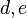# Difference between revisions of "RSA algorithm"

## Summary

RSA is a foundational algorithm of public key cryptography. Any public key cryptography algorithm has the following form: an encryption function$E$ that is publicly accessible (including to eavesdroppers or malicious parties) and a decryption function$D$ that is known to only the party doing decryption, such that$D \circ E$ is the identity map.

The idea is that$D$ should not be easy to deduce from$E$.

The way it is used in practice is as follows: if$A$ wants to send a message to$B$ but their only channel of communication is accessible to$C$. So,$A$ looks up$B$'s public encryption key (accessible to$C$ as well), then sends$B$ the message encrypted using that key, which$B$ decrypts using the decryption key.$C$ has access to the encrypted message and the encryption key, but cannot decrypt because of lack of access to the decryption key.

For a public key cryptography algorithm, the general format of both the encryption and decryption algorithms are known to everybody. The actual encryption algorithm depends additionally on knowledge of a specific number/string called the encryption key$e$ and the actual decryption algorithm depends on knowledge of a decryption key. Another way of putting this is thus that it should not be possible to quickly determine the decryption key from the encryption key. Actually, we want something stronger -- there should be no way to perform decryption based solely on knowledge of the encryption key.

### General idea

RSA relies on the idea (or belief) that the root computation problem in a group does not have any simple reduction to the power computation problem if the order of the group is not known. The idea is as follows:

1. First, come up with a general family of encodings of groups where the basic black-box group operations (multiplication, inverse, identity element, membership testing) are known and can be performed quickly, but the order of the group is known only to the party that constructs the group and is not known to other parties.
2. Second, the party that has constructed a group selects two integers$d,e$ such that$de$ is congruent to the order of the group.$e$, the encryption key is published to all, and$d$ is kept secret.
3. The encryption algorithm (publicly accessible) works as follows on the elements of the group: an element$h$ of the group is encrypted to$h^e$ (i.e., the power computation problem) and an element$g$ of the group is decrypted to$g^d$. (Note: This algorithm works on elements of the group, but combined with standard algorithms, it can be used to encrypt any string).

Here is a summary:

Party Does this party know the order of the group? Can this party access the group encoding and do the group operations Does this party know the encryption key? Does this party know the decryption key? Does this party know the encrypted message? Does this party know the original message?
encrypting party$A$ No Yes, these are necessary for the encryption algorithm Yes, this is necessary for the encryption algorithm No Yes, after performing the encryption Yes, it created the original message
decrypting party$B$ Yes,$B$ created the group Yes Yes,$B$ created the encryption key Yes,$B$ created the decryption key Yes, after receiving it Yes, after receiving and decrypting it
third party$C$ No Yes, because these are publicly available because$A$ needs them Yes, publicly available because$A$ needs it No Yes, because the message is communicated on a channel that$C$ can access No, because$C$ cannot decrypt